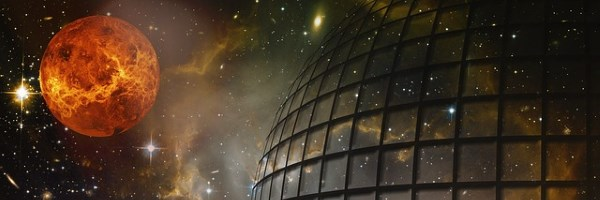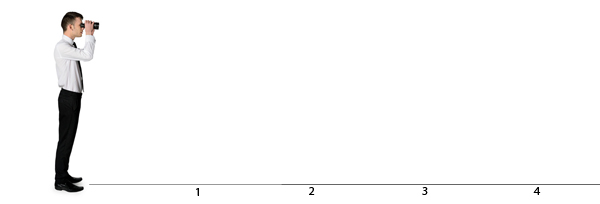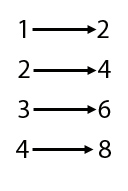# What is infinity?In a sense we all have an inkling of what infinity is. It's something that characterises things that never end. The list of natural numbers 1, 2, 3, 4, ...., for example, or an infinitely long straight line that starts at a point just in front of you and stretches on forever, straight ahead. You can't reach the end of that line even if you travel in the fastest car, and no matter how long you count for, you can never reach the end of the natural numbers.

But are these two types of infinity, that of the natural numbers and that of the line, the same? Intuitively you might think that they are different. The natural numbers are separate "things", while the line is all connected up. You could place the natural numbers along your line, at distance 1 metre apart. This gives a sense that there is somehow more to the infinity of the line than to the infinity of the natural numbers: the line is able to fill the gaps between the numbers.Mathematicians agree with that intuition. They distinguish between countable infinities and uncountable ones. The natural numbers form a countable infinity, and that makes sense, as you could count through all of them if you had an infinite amount of time. A group of infinitely many people also qualifies as a countable infinity. That's because (with an infinite amount of time) you could make a list of all the names, each name taking its own place on the list, and then you could count through them, just as you can count through the natural numbers. In general, an infinite collection of objects forms a countable infinity if you can list the objects one by one, with a place on the list for every object and one object for every place on the list.

What about the infinitely long straight line? It is also made up of infinitely many objects: in this case the objects are points on the line. If you imagine this line as an infinitely long ruler, then each point comes with a number: the starting point of the line comes with the number 0, the point half a metre along comes with the number 0.5, and so on (the collection of numbers you get from the ruler are called the positive real numbers). Can you make a list of those numbers to show that they also form a countable infinity?

One approach would be to order those numbers by size. But that quickly gets you into trouble. Clearly the first number should be 0, but what about the second one? You could try 0.1, but then, 0.01 is smaller than that, so it should come before 0.1. But what about 0.001? For every number you might designate as taking the second place on the list you can find a smaller one (you simply insert an extra 0 after the decimal point). So listing those numbers along the ruler by size is hopeless.

Could there be another way of listing them? The answer is no. There is a mathematical argument (see here) which shows that any list of positive real numbers definitely misses out at least one other positive real number. You can never make a complete list. This shows that the infinity represented by the infinite straight line (or, equivalently, the positive real numbers) is an uncountable infinity.

### Which infinity is bigger?

What about the idea that the infinity of the infinite line is somehow "bigger" than the infinity of the natural numbers? One way of comparing the size of finite collections of things, if you can't be bothered to count, is to see if you can match them up exactly. Think of a number of chairs and a number of people. If there is a chair for every person and no chairs are left over, then you know that there must be the same number of chairs as people. If there are chairs left over, then you know that there are more chairs than people. And if there are some people left standing, you know that there are more people than chairs.

You can extend this idea to infinite collections of objects. If you can match the objects in collection A to the objects in collection B exactly, with every object in A corresponding to exactly one object in B and vice versa, then we say that the two collection have the same size or, as mathematicians put it, the same cardinality. We have already seen this in action with our infinite group of people above. By listing them one by one we have actually matched them up exactly with the natural numbers: for every person there is exactly one natural number (their place on the list) and for every natural number there is exactly one person (the one occupying the place on the list given by that natural number). This is why we say that the group of people and the natural numbers represent the same kind of infinity — a countable one.

Going back to points on the infinite line, however, it turns out that any attempt to list them (to match them exactly with the natural numbers) misses out at least one point. This is why we say that the cardinality of the line (an uncountable infinity) is greater than the cardinality of the natural numbers (a countable infinity).

### Countable confusion

Intuitively, uncountable infinities appear more unwieldy and tricky than countable ones and in mathematics they often are. But this doesn't mean that countable ones are straight-forward. As an example, think of all the even numbers 2, 4, 6, 8, and so on. There are infinitely many, but what is the cardinality ("size") of that infinity compared to that of all the natural numbers? Surely it should be half as big?

The answer is no. We said that two infinite collections have the same cardinality if the objects in one can be matched up exactly with the object in the other. It's pretty easy to match all the even numbers exactly with all the natural numbers:So the cardinality of the even numbers is the same as that of the natural ones. If this seems weird then perhaps the next result is even weirder. It's possible to show (see here) that all the rational numbers (that is, all the fractions such as 1/2 or 5/6) can also be listed; that is, they can be matched exactly with the natural numbers. So even though there appear to be many more fractions than natural numbers (there are infinitely many fractions between any two successive natural numbers) the two sets of numbers have the same cardinality.

The eminent Galileo Galilei discovered such strange facts about infinity back in the 17th century and thought they were so weird, it put him off thinking about infinity claiming that "we cannot speak of infinite quantities as being the one greater or less than or equal to another". Over 200 years later, the mathematician Georg Cantor picked those ideas up again, undeterred by their weirdness, and went much further. He discovered a whole tower of infinities, each one larger than the other. The infinities of the natural numbers and the infinity of the line are only two of them.